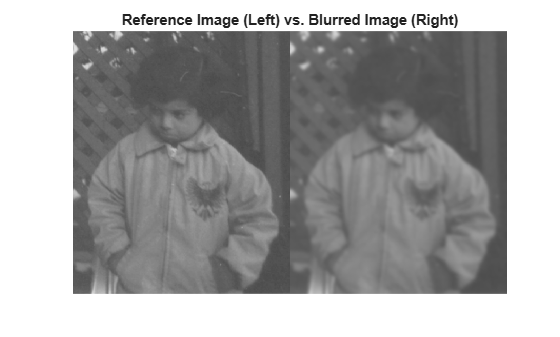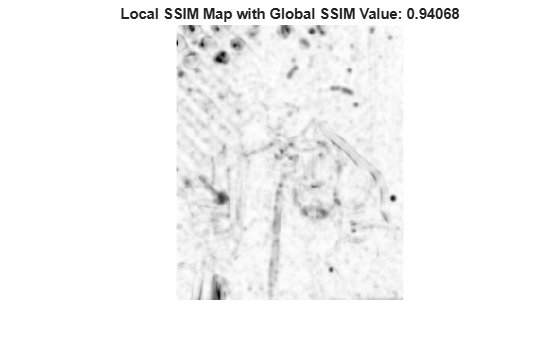# ssim

Structural similarity (SSIM) index for measuring image quality

## Syntax

``ssimval = ssim(A,ref)``
``ssimval = ssim(A,ref,Name,Value)``
``````[ssimval,ssimmap] = ssim(___)``````

## Description

example

````ssimval = ssim(A,ref)` calculates the structural similarity (SSIM) index for grayscale image or volume `A` using `ref` as the reference image or volume. A value closer to 1 indicates better image quality.```
````ssimval = ssim(A,ref,Name,Value)` calculates the SSIM, using name-value pairs to control aspects of the computation.```
``````[ssimval,ssimmap] = ssim(___)``` also returns the local SSIM value for each pixel or voxel in `A`. ```

## Examples

collapse all

Read an image into the workspace. Create another version of the image, applying a blurring filter.

```ref = imread("pout.tif"); H = fspecial("Gaussian",[11 11],1.5); A = imfilter(ref,H,"replicate");```

Display both images as a montage. The images differ most along sharp high-contrast regions, such as the edges of the trellis.

```montage({ref,A}) title("Reference Image (Left) vs. Blurred Image (Right)")```Calculate the global SSIM value for the image and local SSIM values for each pixel.

`[ssimval,ssimmap] = ssim(A,ref);`

Display the local SSIM map. Include the global SSIM value in the figure title. Small values of local SSIM appear as dark pixels in the local SSIM map. Regions with small local SSIM value correspond to areas where the blurred image noticeably differs from the reference image. Large values of local SSIM value appear as bright pixels. Regions with large local SSIM correspond to uniform regions of the reference image, where blurring has less of an impact on the image.

```imshow(ssimmap,[]) title("Local SSIM Map with Global SSIM Value: "+num2str(ssimval))```Read an image into the workspace. Create another version of the image, applying a blurring filter.

```ref = imread("pout.tif"); A = imgaussfilt(ref,1.5,"FilterSize",11,"Padding","replicate");```

Display both images as a montage.

```montage({ref A}) title("Reference Image (Left) vs. Blurred Image (Right)")```Simulate batches of images by replicating the reference image and the blurred image 16 times along the 4th dimension.

```A = repmat(A,[1 1 1 16]); ref = repmat(ref,[1 1 1 16]);```

Create formatted `dlarray` objects for the reference image batch and the blurred image batch. The format is "`SSCB`", for spatial-spatial-channel-batch.

```A = dlarray(single(A),"SSCB"); ref = dlarray(single(ref),"SSCB");```

Calculate the global SSIM value for the image and local SSIM values for each pixel. `ssimVal` returns a scalar SSIM value for each image in the batch. `ssimMap` returns a map of SSIM values, the same size as the image, for each image in the batch. ` `

```[ssimVal,ssimMap] = ssim(A,ref); size(ssimVal)```
```ans = 1×4 1 1 1 16 ```
`size(ssimMap)`
```ans = 1×4 291 240 1 16 ```

## Input Arguments

collapse all

Image for quality measurement, specified as a numeric array or a `dlarray` (Deep Learning Toolbox) object. If `A` is not a 2-D grayscale image or 3-D grayscale volume, such as an RGB image or stack of grayscale images, specify the `DataFormat` name-value argument. Do not specify the `DataFormat` name-value argument if `A` is a formatted `dlarray` object.

Data Types: `single` | `double` | `int16` | `uint8` | `uint16`

Reference image against which to measure quality, specified as a numeric array or a `dlarray` (Deep Learning Toolbox) object of the same size and data type as `A`. If `ref` is not a 2-D grayscale image or 3-D grayscale volume, such as an RGB image or stack of grayscale images, specify the `DataFormat` name-value argument. Do not specify the `DataFormat` name-value argument if `ref` is a formatted `dlarray` object.

Data Types: `single` | `double` | `int16` | `uint8` | `uint16`

### Name-Value Arguments

Specify optional pairs of arguments as `Name1=Value1,...,NameN=ValueN`, where `Name` is the argument name and `Value` is the corresponding value. Name-value arguments must appear after other arguments, but the order of the pairs does not matter.

Before R2021a, use commas to separate each name and value, and enclose `Name` in quotes.

Example: `ssim(A,ref,"DynamicRange",100)`

Dimension labels of the input images `A` and `ref`, specified as a string scalar or character vector. Each character in `DataFormat` must be one of these labels:

• `S` — Spatial

• `C` — Channel

• `B` — Batch observations

The format cannot include more than one channel label or batch label. Do not specify the `DataFormat` name-value argument when the input images are formatted `dlarray` objects.

Example: `"SSC"` indicates that the array has two spatial dimensions and one channel dimension, appropriate for 2-D RGB image data.

Example: `"SSCB"` indicates that the array has two spatial dimensions, one channel dimension, and one batch dimension, appropriate for a sequence of 2-D RGB image data.

Data Types: `char` | `string`

Dynamic range of the input image, specified as a positive scalar. The default value of `"DynamicRange"` depends on the data type of image `A`, and is calculated as `diff(getrangefromclass(A))`. For example, the default dynamic range is `255` for images of data type `uint8`, and the default is `1` for images of data type `double` or `single` with pixel values in the range [0, 1].

Data Types: `single` | `double` | `int8` | `int16` | `int32` | `uint8` | `uint16` | `uint32`

Exponents for the luminance, contrast, and structural terms, specified as a 3-element vector of nonnegative numbers of the form ```[alpha beta gamma]```.

Data Types: `single` | `double` | `int8` | `int16` | `int32` | `uint8` | `uint16` | `uint32`

Standard deviation of isotropic Gaussian function, specified as a positive number. This value is used for weighting the neighborhood pixels around a pixel for estimating local statistics. This weighting is used to avoid blocking artifacts in estimating local statistics.

Data Types: `single` | `double` | `int8` | `int16` | `int32` | `uint8` | `uint16` | `uint32`

Regularization constants for the luminance, contrast, and structural terms, specified as a 3-element vector of nonnegative numbers of the form `[c1 c2 c3]`. The `ssim` function uses these regularization constants to avoid instability for image regions where the local mean or standard deviation is close to zero. Therefore, small non-zero values should be used for these constants.

By default,

• `C1 = (0.01*L).^2`, where `L` is the specified `DynamicRange` value.

• `C2 = (0.03*L).^2`, where `L` is the specified `DynamicRange` value.

• `C3 = C2/2`

Data Types: `single` | `double` | `int8` | `int16` | `int32` | `uint8` | `uint16` | `uint32`

## Output Arguments

collapse all

SSIM index, returned as one of these values.

Input Image TypeSSIM Value

• Unformatted numeric arrays

• Formatted numeric arrays with neither a channel (`"C"`) nor batch (`"B"`) dimension

Numeric scalar with a single SSIM measurement.

Scalar `dlarray` object with a single SSIM measurement.

• Numeric arrays with a channel or batch dimension specified using the `DataFormat` name-value argument

Numeric array of the same dimensionality as the input images. The spatial dimensions of `ssimval` are singleton dimensions. There is one SSIM measurement for each element along any channel or batch dimension.
• Formatted `dlarray` objects with a channel or batch dimension

• Unformatted `dlarray` objects with a channel or batch dimension specified using the `DataFormat` name-value argument

`dlarray` object of the same dimensionality as the input images. The spatial dimensions of `ssimval` are singleton dimensions. There is one SSIM measurement for each element along any channel or batch dimension.

`ssimval` is of data type `double` except when `A` is of data type `single`, in which case `ssimval` is of data type `single`.

The value of `ssimval` is typically in the range [0, 1]. The value 1 indicates the highest quality and occurs when `A` and `ref` are equivalent. Smaller values correspond to poorer quality. For some combinations of inputs and name-value pair arguments, `ssimval` can be negative.

Local values of the SSIM index, returned as one of these values.

Input Image TypeSSIM Value

• Unformatted numeric arrays

• Formatted numeric arrays with neither a channel (`"C"`) nor batch (`"B"`) dimension

Numeric array the same size as the input images. There is one SSIM measurement for each element in the input image.
`dlarray` object the same size as the input images. There is one SSIM measurement for each element in the input image.
• Numeric arrays with a channel or batch dimension specified using the `DataFormat` name-value argument

Numeric array the same size as the input images. Each spatial element in the input image has an SSIM measurement along any channel or batch dimension.
• Formatted `dlarray` objects with a channel or batch dimension

• Unformatted `dlarray` objects with a channel or batch dimension specified using the `DataFormat` name-value argument

`dlarray` object the same size as the input images. Each spatial element in the input image has an SSIM measurement along any channel or batch dimension.

`ssimmap` is of data type `double` except when `A` is of data type `single`, in which case `ssimmap` is of data type `single`.

collapse all

### Structural Similarity Index

An image quality metric that assesses the visual impact of three characteristics of an image: luminance, contrast and structure.

## Algorithms

The SSIM Index quality assessment index is based on the computation of three terms, namely the luminance term, the contrast term and the structural term. The overall index is a multiplicative combination of the three terms.

`$SSIM\left(x,y\right)={\left[l\left(x,y\right)\right]}^{\alpha }\cdot {\left[c\left(x,y\right)\right]}^{\beta }\cdot {\left[s\left(x,y\right)\right]}^{\gamma }$`

where

`$\begin{array}{l}l\left(x,y\right)=\frac{2{\mu }_{x}{\mu }_{y}+{C}_{1}}{{\mu }_{x}^{2}+{\mu }_{y}^{2}+{C}_{1}},\\ c\left(x,y\right)=\frac{2{\sigma }_{x}{\sigma }_{y}+{C}_{2}}{{\sigma }_{x}^{2}+{\sigma }_{y}^{2}+{C}_{2}},\\ s\left(x,y\right)=\frac{{\sigma }_{xy}+{C}_{3}}{{\sigma }_{x}{\sigma }_{y}+{C}_{3}}\end{array}$`

where μx, μy, σxy, and σxy are the local means, standard deviations, and cross-covariance for images x, y. If α = β = γ = 1 (the default for `Exponents`), and C3 = C2/2 (default selection of C3) the index simplifies to:

`$SSIM\left(x,y\right)=\frac{\left(2{\mu }_{x}{\mu }_{y}+{C}_{1}\right)\left(2{\sigma }_{xy}+{C}_{2}\right)}{\left({\mu }_{x}^{2}+{\mu }_{y}^{2}+{C}_{1}\right)\left({\sigma }_{x}^{2}+{\sigma }_{y}^{2}+{C}_{2}\right)}$`

When you specify a noninteger value for `"Exponents"`, the `ssim` function prevents complex valued outputs by clamping the intermediate luminance, contrast, and structural terms to the range [0, `inf`].

 Zhou, W., A. C. Bovik, H. R. Sheikh, and E. P. Simoncelli. "Image Quality Assessment: From Error Visibility to Structural Similarity." IEEE Transactions on Image Processing. Vol. 13, Issue 4, April 2004, pp. 600–612.